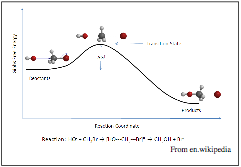CH302H - Principles of Chemistry II: Honors Spring 2014, Unique 51880 Homework, Week 14

 Bonus Quiz Homework, week 14 Answer Key Homework week 14 (more kinetics)   1. Gases adsorb to surfaces to differing degrees: physisorption (weak) to chemisorption (strong). Sometimes they exhibit dissociative adsorption, such as the case of O2 adsorbing onto carbon that we discussed in class. Often physisorbed molecules freely move about on the surface, i.e., said to “skate” on the surface.   Desorption involves the removal (“vaporization”) of these adsorbed species. The enthalpy of desorption needed to remove chemisorbed molecules that are not dissociatively adsorbed is obviously larger than for physisorbed molecules. However, the temperatures at which significant desorption rates begin are not as different as one would expect based on their  differences in ∆H. Using the equation describing the rate constant, k, for transition state theory, explain why this might be the case. (Obviously, the frequency factor, A, must be significantly different, but why?) 2.a) Shown above is a graph illustrating the concept of transition state theory. How does the presence of a catalyst impact the graph?   b) Is ∆Go, ∆So or ∆Ho for the reaction affected by the catalyst’s presence?   c) Why are enzymes needed in living organisms in order to convert glucose to energy? After all, the reaction is spontaneous (∆Go << 0).  d) Looking at the complete (and somewhat complex) equations describing k using collision theory and k using transition state theory: What parameter(s) is(are) likely impacted by a catalyst?   3. How much faster would a reaction proceed if the temperature were raised from 298K to 340K, given an activation energy of 50. kJ/mole?   4. Atop a mountain where water boils at 92oC, a “3 minute egg” prepared at sea level requires 5 minutes to prepare. Estimate the activation energy for cooking the egg. (The “cooking” in this instance is protein denaturation.)   5. A general kinetic axiom is that rates double for every increase of 10oC in temperature. Assuming a starting temperature of 25oC, what is the activation energy assumed when making such a statement?